JEE  >  NCERT Solutions Exercise 5.7: Continuity & Differentiability

# NCERT Solutions Exercise 5.7: Continuity & Differentiability | Mathematics For JEE

 1 Crore+ students have signed up on EduRev. Have you?

Continuity & Differentiability

Question 1: Find the second order derivatives of the function.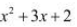Let y =Then,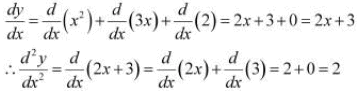Question 2: Find the second order derivatives of the function. x20

Let y = x20
Then,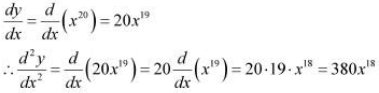Question 3: Find the second order derivatives of the function. x.cos x

Let y = x.cos x
Then,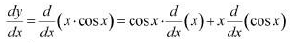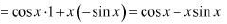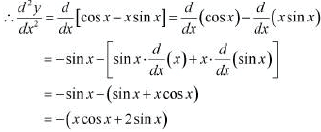Question 4: Find the second order derivatives of the function. log x

Let y = log x
Then,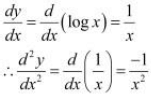Question 5: Find the second order derivatives of the function. x3 log x

Let y = x3 log x
Then,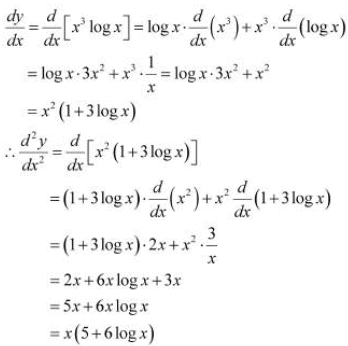Question 6: Find the second order derivatives of the function.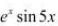Let y =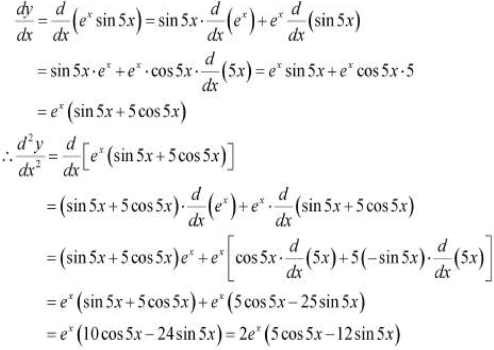Question 7: Find the second order derivatives of the function.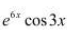Let y =Then,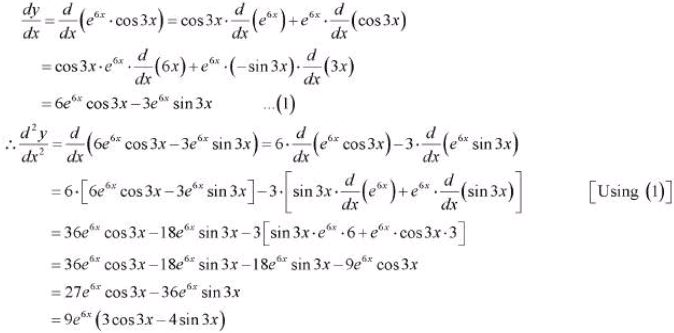Question 8: Find the second order derivatives of the function.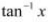Let y =Then,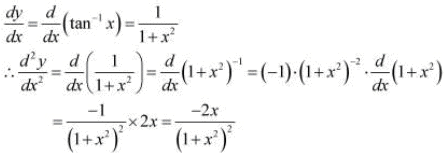Question 9: Find the second order derivatives of the function.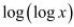Let y =Then,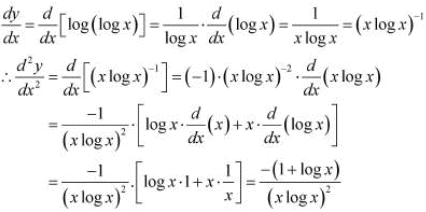Question 10: Find the second order derivatives of the function.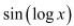Let y =Then,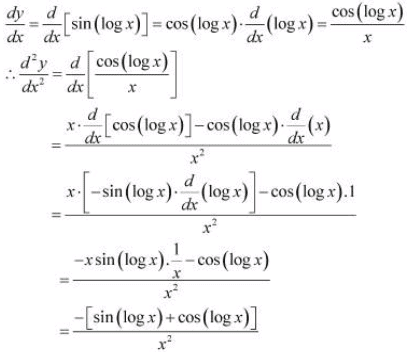Question 11: If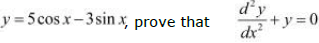It is given that,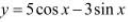Then,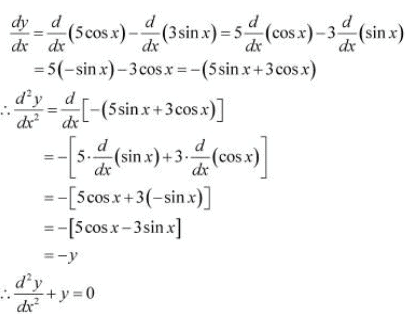Hence, proved.

Question 12:
If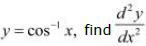in terms of y alone.

It is given that,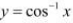Then,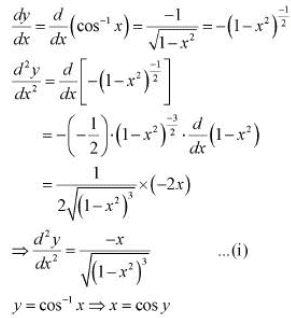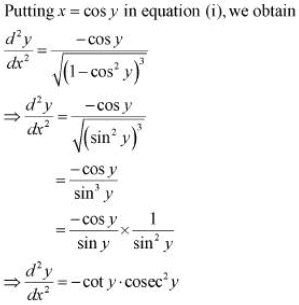Question 13:
If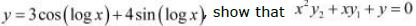It is given that,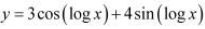Then,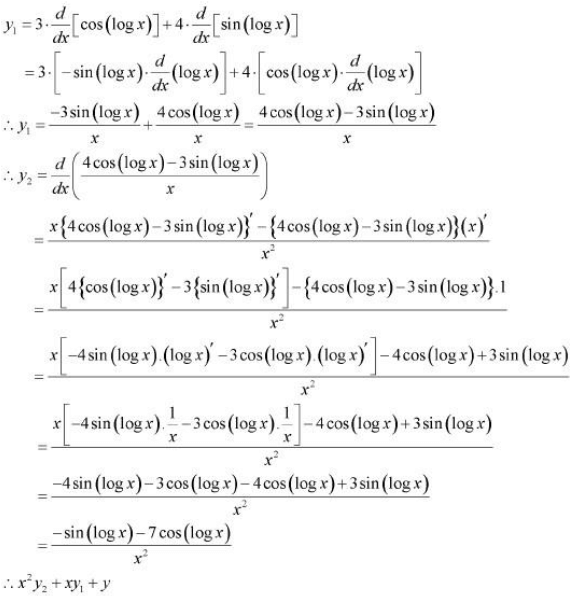Hence, proved.

Question 14:
If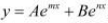, show that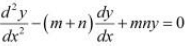It is given that,Then,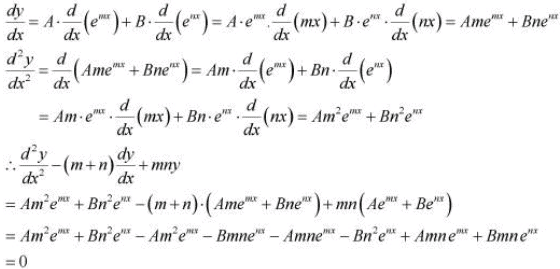Hence, proved.

Question 15:
If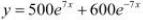, show that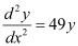It is given that,Then,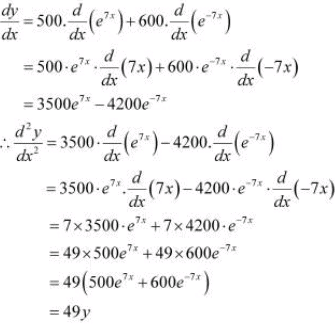Hence, proved.

Question 16:
If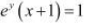, show that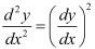The given relationship is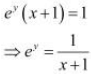Taking logarithm on both the sides, we obtain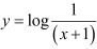Differentiating this relationship with respect to x, we obtain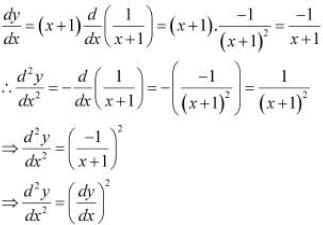Hence, proved.

Question 17:
If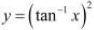, show that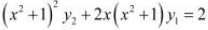The given relationship isThen,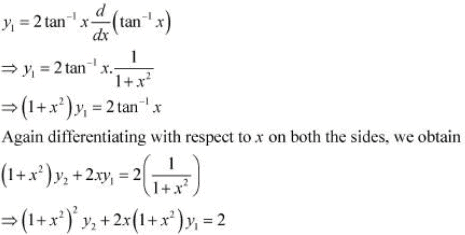Hence, proved.

The document NCERT Solutions Exercise 5.7: Continuity & Differentiability | Mathematics For JEE is a part of the JEE Course Mathematics For JEE.
All you need of JEE at this link: JEE

## Mathematics For JEE

130 videos|359 docs|306 tests

## Mathematics For JEE

130 videos|359 docs|306 tests

Track your progress, build streaks, highlight & save important lessons and more!(Scan QR code)

,

,

,

,

,

,

,

,

,

,

,

,

,

,

,

,

,

,

,

,

,

;